Merge Sort

• Difficulty Level : Medium
• Last Updated : 10 Jan, 2022

Like QuickSort, Merge Sort is a Divide and Conquer algorithm. It divides the input array into two halves, calls itself for the two halves, and then merges the two sorted halves. The merge() function is used for merging two halves. The merge(arr, l, m, r) is a key process that assumes that arr[l..m] and arr[m+1..r] are sorted and merges the two sorted sub-arrays into one. See the following C implementation for details.

MergeSort(arr[], l,  r)
If r > l
1. Find the middle point to divide the array into two halves:
middle m = l+ (r-l)/2
2. Call mergeSort for first half:
Call mergeSort(arr, l, m)
3. Call mergeSort for second half:
Call mergeSort(arr, m+1, r)
4. Merge the two halves sorted in step 2 and 3:
Call merge(arr, l, m, r)

The following diagram from wikipedia shows the complete merge sort process for an example array {38, 27, 43, 3, 9, 82, 10}. If we take a closer look at the diagram, we can see that the array is recursively divided into two halves till the size becomes 1. Once the size becomes 1, the merge processes come into action and start merging arrays back till the complete array is merged.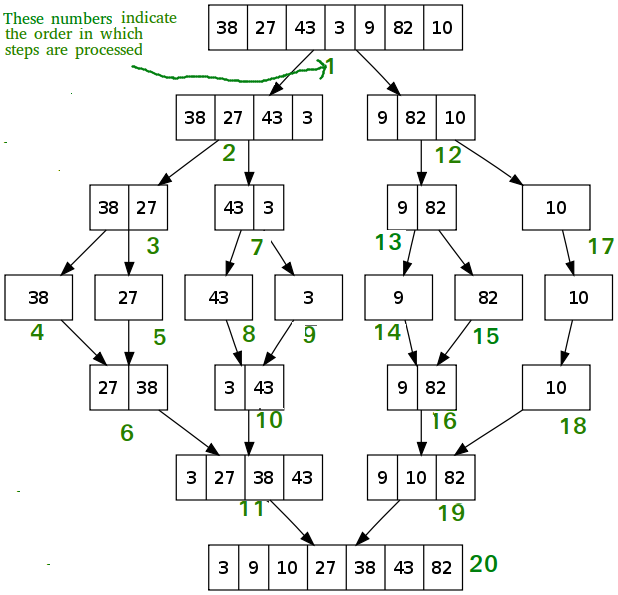C++

 // C++ program for Merge Sort#include using namespace std;  // Merges two subarrays of array[].// First subarray is arr[begin..mid]// Second subarray is arr[mid+1..end]void merge(int array[], int const left, int const mid, int const right){    auto const subArrayOne = mid - left + 1;    auto const subArrayTwo = right - mid;      // Create temp arrays    auto *leftArray = new int[subArrayOne],         *rightArray = new int[subArrayTwo];      // Copy data to temp arrays leftArray[] and rightArray[]    for (auto i = 0; i < subArrayOne; i++)        leftArray[i] = array[left + i];    for (auto j = 0; j < subArrayTwo; j++)        rightArray[j] = array[mid + 1 + j];      auto indexOfSubArrayOne = 0, // Initial index of first sub-array        indexOfSubArrayTwo = 0; // Initial index of second sub-array    int indexOfMergedArray = left; // Initial index of merged array      // Merge the temp arrays back into array[left..right]    while (indexOfSubArrayOne < subArrayOne && indexOfSubArrayTwo < subArrayTwo) {        if (leftArray[indexOfSubArrayOne] <= rightArray[indexOfSubArrayTwo]) {            array[indexOfMergedArray] = leftArray[indexOfSubArrayOne];            indexOfSubArrayOne++;        }        else {            array[indexOfMergedArray] = rightArray[indexOfSubArrayTwo];            indexOfSubArrayTwo++;        }        indexOfMergedArray++;    }    // Copy the remaining elements of    // left[], if there are any    while (indexOfSubArrayOne < subArrayOne) {        array[indexOfMergedArray] = leftArray[indexOfSubArrayOne];        indexOfSubArrayOne++;        indexOfMergedArray++;    }    // Copy the remaining elements of    // right[], if there are any    while (indexOfSubArrayTwo < subArrayTwo) {        array[indexOfMergedArray] = rightArray[indexOfSubArrayTwo];        indexOfSubArrayTwo++;        indexOfMergedArray++;    }}  // begin is for left index and end is// right index of the sub-array// of arr to be sorted */void mergeSort(int array[], int const begin, int const end){    if (begin >= end)        return; // Returns recursively      auto mid = begin + (end - begin) / 2;    mergeSort(array, begin, mid);    mergeSort(array, mid + 1, end);    merge(array, begin, mid, end);}  // UTILITY FUNCTIONS// Function to print an arrayvoid printArray(int A[], int size){    for (auto i = 0; i < size; i++)        cout << A[i] << " ";}  // Driver codeint main(){    int arr[] = { 12, 11, 13, 5, 6, 7 };    auto arr_size = sizeof(arr) / sizeof(arr);      cout << "Given array is \n";    printArray(arr, arr_size);      mergeSort(arr, 0, arr_size - 1);      cout << "\nSorted array is \n";    printArray(arr, arr_size);    return 0;}  // This code is contributed by Mayank Tyagi// This code was revised by Joshua Estes

C

 /* C program for Merge Sort */#include #include   // Merges two subarrays of arr[].// First subarray is arr[l..m]// Second subarray is arr[m+1..r]void merge(int arr[], int l, int m, int r){    int i, j, k;    int n1 = m - l + 1;    int n2 = r - m;      /* create temp arrays */    int L[n1], R[n2];      /* Copy data to temp arrays L[] and R[] */    for (i = 0; i < n1; i++)        L[i] = arr[l + i];    for (j = 0; j < n2; j++)        R[j] = arr[m + 1 + j];      /* Merge the temp arrays back into arr[l..r]*/    i = 0; // Initial index of first subarray    j = 0; // Initial index of second subarray    k = l; // Initial index of merged subarray    while (i < n1 && j < n2) {        if (L[i] <= R[j]) {            arr[k] = L[i];            i++;        }        else {            arr[k] = R[j];            j++;        }        k++;    }      /* Copy the remaining elements of L[], if there    are any */    while (i < n1) {        arr[k] = L[i];        i++;        k++;    }      /* Copy the remaining elements of R[], if there    are any */    while (j < n2) {        arr[k] = R[j];        j++;        k++;    }}  /* l is for left index and r is right index of thesub-array of arr to be sorted */void mergeSort(int arr[], int l, int r){    if (l < r) {        // Same as (l+r)/2, but avoids overflow for        // large l and h        int m = l + (r - l) / 2;          // Sort first and second halves        mergeSort(arr, l, m);        mergeSort(arr, m + 1, r);          merge(arr, l, m, r);    }}  /* UTILITY FUNCTIONS *//* Function to print an array */void printArray(int A[], int size){    int i;    for (i = 0; i < size; i++)        printf("%d ", A[i]);    printf("\n");}  /* Driver code */int main(){    int arr[] = { 12, 11, 13, 5, 6, 7 };    int arr_size = sizeof(arr) / sizeof(arr);      printf("Given array is \n");    printArray(arr, arr_size);      mergeSort(arr, 0, arr_size - 1);      printf("\nSorted array is \n");    printArray(arr, arr_size);    return 0;}

Java

 /* Java program for Merge Sort */class MergeSort {    // Merges two subarrays of arr[].    // First subarray is arr[l..m]    // Second subarray is arr[m+1..r]    void merge(int arr[], int l, int m, int r)    {        // Find sizes of two subarrays to be merged        int n1 = m - l + 1;        int n2 = r - m;          /* Create temp arrays */        int L[] = new int[n1];        int R[] = new int[n2];          /*Copy data to temp arrays*/        for (int i = 0; i < n1; ++i)            L[i] = arr[l + i];        for (int j = 0; j < n2; ++j)            R[j] = arr[m + 1 + j];          /* Merge the temp arrays */          // Initial indexes of first and second subarrays        int i = 0, j = 0;          // Initial index of merged subarray array        int k = l;        while (i < n1 && j < n2) {            if (L[i] <= R[j]) {                arr[k] = L[i];                i++;            }            else {                arr[k] = R[j];                j++;            }            k++;        }          /* Copy remaining elements of L[] if any */        while (i < n1) {            arr[k] = L[i];            i++;            k++;        }          /* Copy remaining elements of R[] if any */        while (j < n2) {            arr[k] = R[j];            j++;            k++;        }    }      // Main function that sorts arr[l..r] using    // merge()    void sort(int arr[], int l, int r)    {        if (l < r) {            // Find the middle point            int m =l+ (r-l)/2;              // Sort first and second halves            sort(arr, l, m);            sort(arr, m + 1, r);              // Merge the sorted halves            merge(arr, l, m, r);        }    }      /* A utility function to print array of size n */    static void printArray(int arr[])    {        int n = arr.length;        for (int i = 0; i < n; ++i)            System.out.print(arr[i] + " ");        System.out.println();    }      // Driver code    public static void main(String args[])    {        int arr[] = { 12, 11, 13, 5, 6, 7 };          System.out.println("Given Array");        printArray(arr);          MergeSort ob = new MergeSort();        ob.sort(arr, 0, arr.length - 1);          System.out.println("\nSorted array");        printArray(arr);    }}/* This code is contributed by Rajat Mishra */

Python3

 # Python program for implementation of MergeSortdef mergeSort(arr):    if len(arr) > 1:           # Finding the mid of the array        mid = len(arr)//2          # Dividing the array elements        L = arr[:mid]          # into 2 halves        R = arr[mid:]          # Sorting the first half        mergeSort(L)          # Sorting the second half        mergeSort(R)          i = j = k = 0          # Copy data to temp arrays L[] and R[]        while i < len(L) and j < len(R):            if L[i] < R[j]:                arr[k] = L[i]                i += 1            else:                arr[k] = R[j]                j += 1            k += 1          # Checking if any element was left        while i < len(L):            arr[k] = L[i]            i += 1            k += 1          while j < len(R):            arr[k] = R[j]            j += 1            k += 1  # Code to print the list    def printList(arr):    for i in range(len(arr)):        print(arr[i], end=" ")    print()    # Driver Codeif __name__ == '__main__':    arr = [12, 11, 13, 5, 6, 7]    print("Given array is", end="\n")    printList(arr)    mergeSort(arr)    print("Sorted array is: ", end="\n")    printList(arr)  # This code is contributed by Mayank Khanna

C#

 // C# program for Merge Sortusing System;class MergeSort {      // Merges two subarrays of []arr.    // First subarray is arr[l..m]    // Second subarray is arr[m+1..r]    void merge(int[] arr, int l, int m, int r)    {        // Find sizes of two        // subarrays to be merged        int n1 = m - l + 1;        int n2 = r - m;          // Create temp arrays        int[] L = new int[n1];        int[] R = new int[n2];        int i, j;          // Copy data to temp arrays        for (i = 0; i < n1; ++i)            L[i] = arr[l + i];        for (j = 0; j < n2; ++j)            R[j] = arr[m + 1 + j];          // Merge the temp arrays          // Initial indexes of first        // and second subarrays        i = 0;        j = 0;          // Initial index of merged        // subarray array        int k = l;        while (i < n1 && j < n2) {            if (L[i] <= R[j]) {                arr[k] = L[i];                i++;            }            else {                arr[k] = R[j];                j++;            }            k++;        }          // Copy remaining elements        // of L[] if any        while (i < n1) {            arr[k] = L[i];            i++;            k++;        }          // Copy remaining elements        // of R[] if any        while (j < n2) {            arr[k] = R[j];            j++;            k++;        }    }      // Main function that    // sorts arr[l..r] using    // merge()    void sort(int[] arr, int l, int r)    {        if (l < r) {            // Find the middle            // point            int m = l+ (r-l)/2;              // Sort first and            // second halves            sort(arr, l, m);            sort(arr, m + 1, r);              // Merge the sorted halves            merge(arr, l, m, r);        }    }      // A utility function to    // print array of size n */    static void printArray(int[] arr)    {        int n = arr.Length;        for (int i = 0; i < n; ++i)            Console.Write(arr[i] + " ");        Console.WriteLine();    }      // Driver code    public static void Main(String[] args)    {        int[] arr = { 12, 11, 13, 5, 6, 7 };        Console.WriteLine("Given Array");        printArray(arr);        MergeSort ob = new MergeSort();        ob.sort(arr, 0, arr.Length - 1);        Console.WriteLine("\nSorted array");        printArray(arr);    }}  // This code is contributed by Princi Singh

Javascript


Output
Given array is
12 11 13 5 6 7
Sorted array is
5 6 7 11 12 13

Time Complexity: Sorting arrays on different machines. Merge Sort is a recursive algorithm and time complexity can be expressed as following recurrence relation.
T(n) = 2T(n/2) + θ(n)

The above recurrence can be solved either using the Recurrence Tree method or the Master method. It falls in case II of Master Method and the solution of the recurrence is θ(nLogn). Time complexity of Merge Sort is  θ(nLogn) in all 3 cases (worst, average and best) as merge sort always divides the array into two halves and takes linear time to merge two halves.
Auxiliary Space: O(n)
Sorting In Place: No in a typical implementation
Stable: Yes

Applications of Merge Sort

1. Merge Sort is useful for sorting linked lists in O(nLogn) time. In the case of linked lists, the case is different mainly due to the difference in memory allocation of arrays and linked lists. Unlike arrays, linked list nodes may not be adjacent in memory. Unlike an array, in the linked list, we can insert items in the middle in O(1) extra space and O(1) time. Therefore, the merge operation of merge sort can be implemented without extra space for linked lists.
In arrays, we can do random access as elements are contiguous in memory. Let us say we have an integer (4-byte) array A and let the address of A be x then to access A[i], we can directly access the memory at (x + i*4). Unlike arrays, we can not do random access in the linked list. Quick Sort requires a lot of this kind of access. In a linked list to access i’th index, we have to travel each and every node from the head to i’th node as we don’t have a continuous block of memory. Therefore, the overhead increases for quicksort. Merge sort accesses data sequentially and the need of random access is low.
2. Inversion Count Problem
3. Used in External Sorting

Drawbacks of Merge Sort

• Slower comparative to the other sort algorithms for smaller tasks.
• Merge sort algorithm requires an additional memory space of 0(n) for the temporary array.
• It goes through the whole process even if the array is sorted.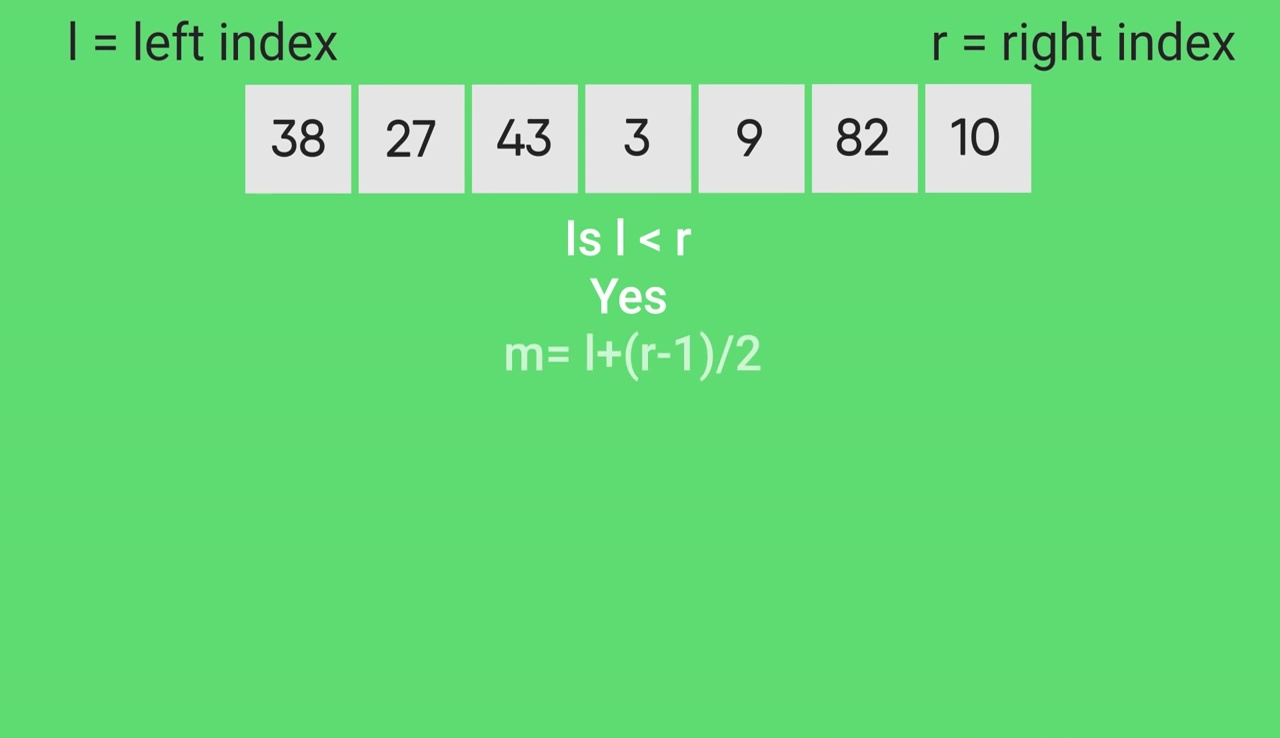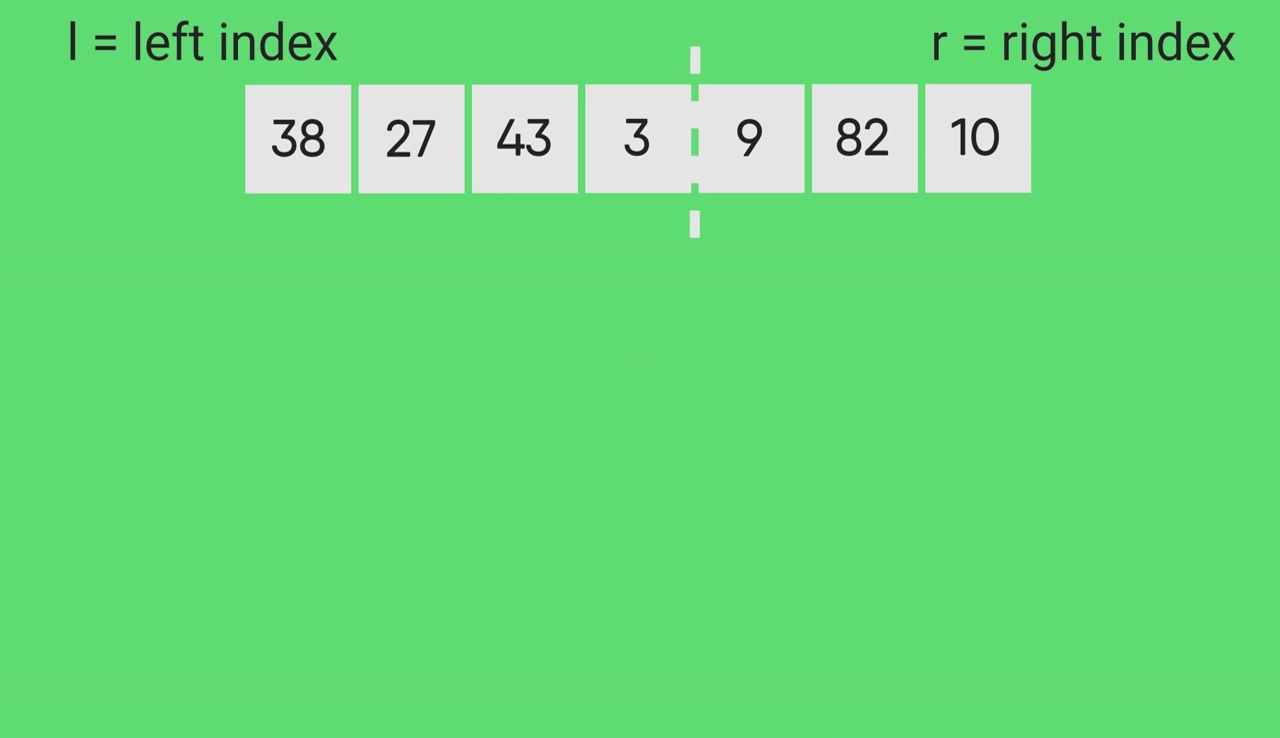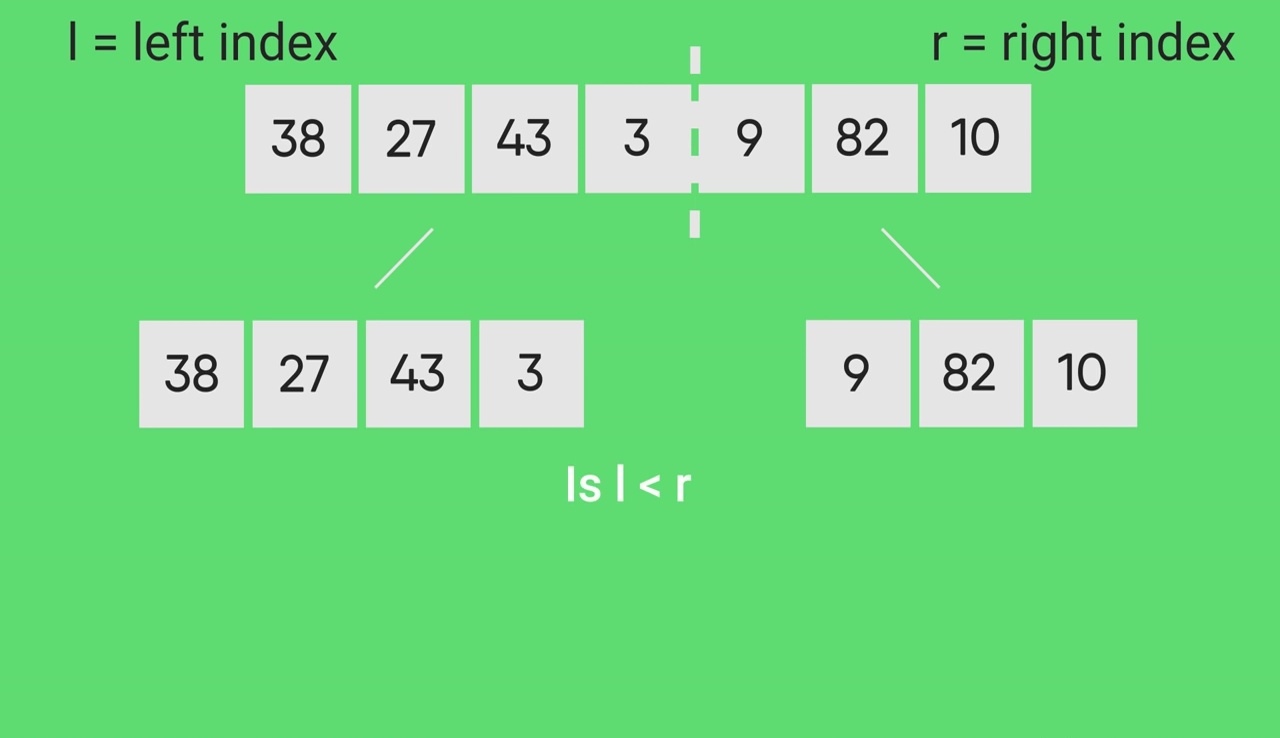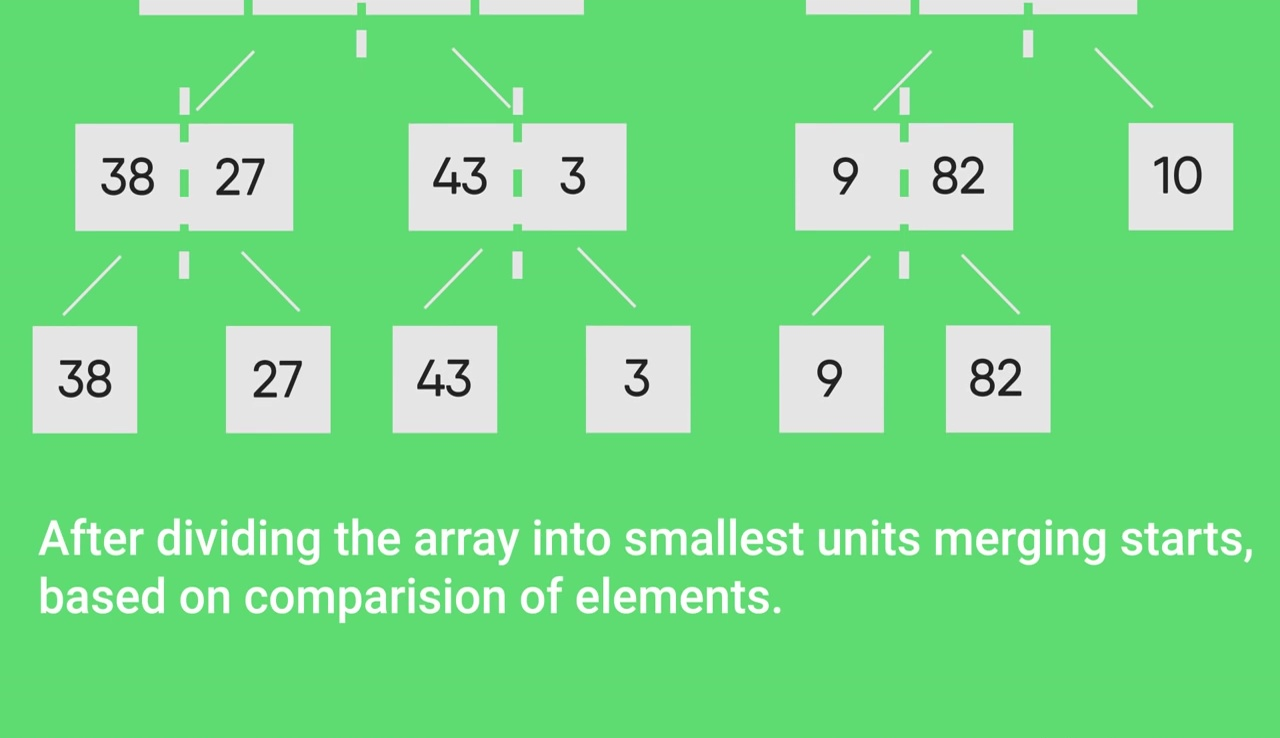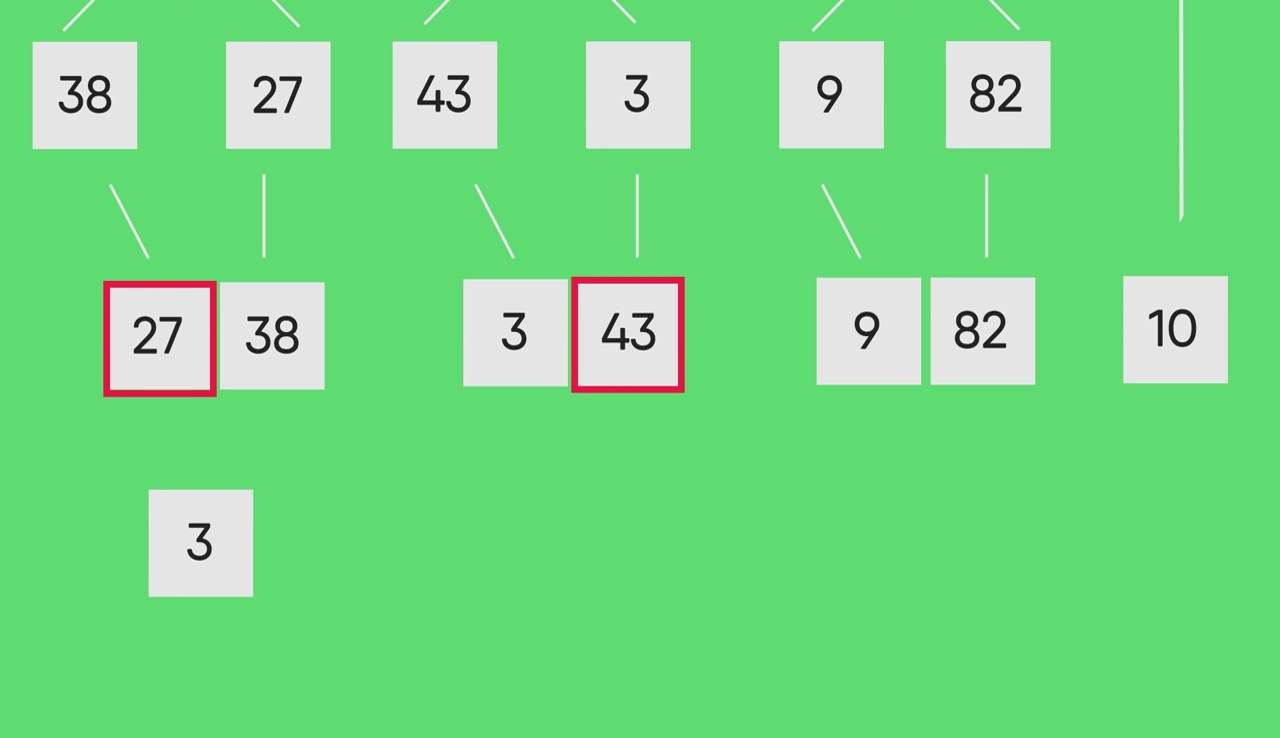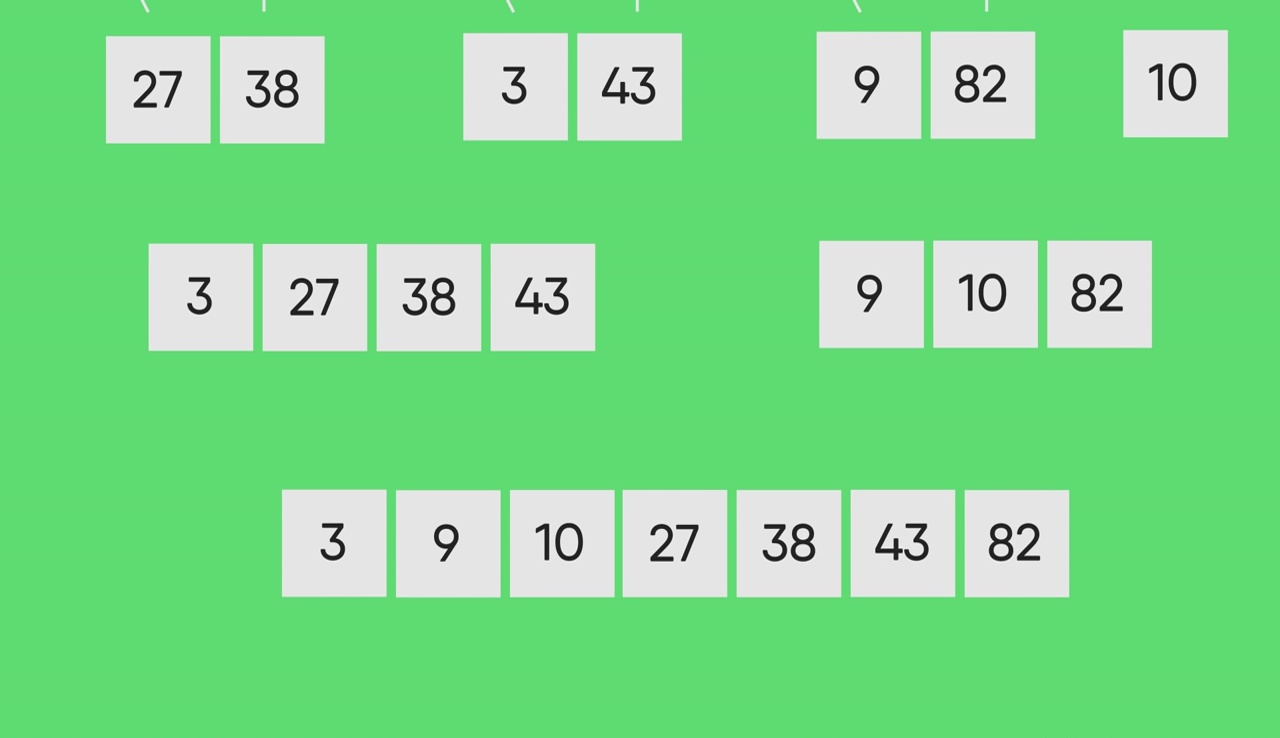Other Sorting Algorithms on GeeksforGeeks:
3-way Merge Sort, Selection Sort, Bubble Sort, Insertion Sort, Merge Sort, Heap Sort, QuickSort, Radix Sort, Counting Sort, Bucket Sort, ShellSort, Comb Sort